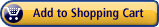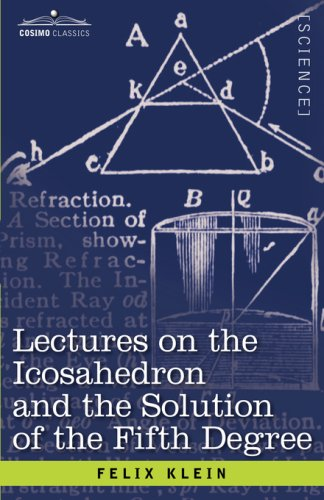Lectures on the Icosahedron and the Solution of the Fifth Degree
by Felix Klein

Shipping on orders over \$25. DetailsAvailability: No info
Ships from and sold by Amazon.com

Editorial Review
 In this classic of mathematical literature, first published in 1884, Felix Klein elegantly demonstrates how the rotation of icosahedron can be used to solve complex quintic equations. Divided into two parts-"Theory of the Icosahedron" and "The Theory of Equations of the Fifth Degree"-The Icosahedron covers: . the regular solids and the theory of groups . introduction of (x + iy) . statement and discussion of the fundamental problem, according to the theory of functions . the algebraical character of the fundamental problem . general theorems and survey of the subject . the historical development of the theory of equations of the fifth degree . introduction of geometrical material . the canonical equations of the fifth degree . the problem of the A's and the Jacobian equations of the sixth degree . the general equation of the fifth degree Complete with detailed equations and instructive material, The Icosahedron will be valued by experts in higher mathematics and students of algebra alike. German mathematician FELIX KLEIN (1849-1925) specialized in function theory, group theory, and non-Euclidean geometry. His published works include Elementary Mathematics from an Advanced Standpoint: Arithmetic, Algebra, Analysis; Elementary Mathematics from an Advanced Standpoint: Geometry; and Famous Problems of Elementary Geometry.

Product Details
• Publisher: Cosimo Classics
• ISBN-10: 1-60206-989-1
• ISBN-13: 978-1-60206-989-3
• Amazon.com Sales Rank #2311778
• Published on: December 01, 2007
• Number of items: 1
• Binding: Hardcover
• 308 pages